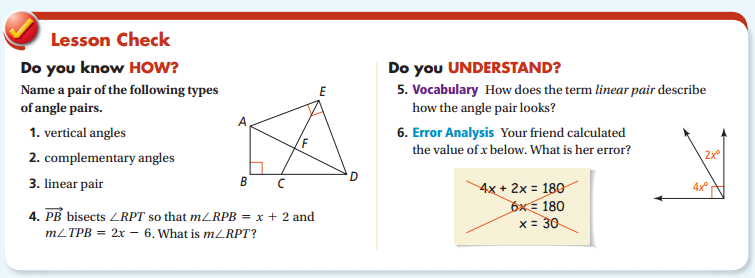# Angle pairs Mathematics Question help

Need to know if all answers are correct.   I have to get 100% on this quiz.  Please check answers and verify they are all correct. Thanks.1.  Vertical angles:  <AFE and <CFD

2.  Complementary angles: <AEF and <DEF

3.  Linear pair: <BCE and <ECD

4.  20

5.  Angles combine to form a straight line

6.  Since it is a right angle instead of straight line, the calculation should be

4x +2x = 90

6x=90

X =15